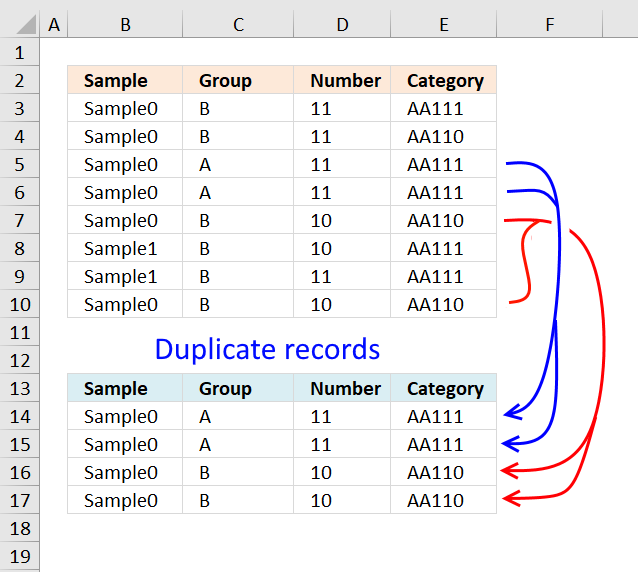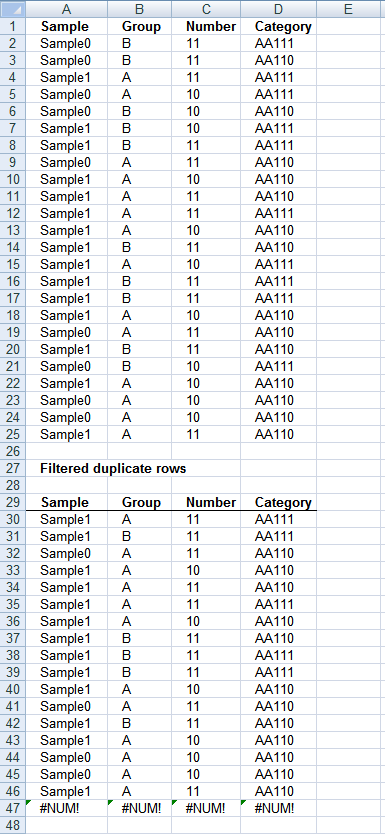Author: Oscar Cronquist Article last updated on February 16, 2018This article describes how to filter duplicate rows with the use of a formula.  It is, in fact, an array formula which is demonstrated below. The function that makes this all possible is the COUNTIFS function, introduced in Excel 2007.

The COUNTIFS function evaluates criteria to cells across multiple ranges and counts the number of times all criteria are met.### Array formula in cell A30:

=INDEX(\$A\$2:\$D\$25, SMALL(IF(COUNTIFS(\$A\$2:\$A\$25, \$A\$2:\$A\$25, \$B\$2:\$B\$25, \$B\$2:\$B\$25, \$C\$2:\$C\$25, \$C\$2:\$C\$25, \$D\$2:\$D\$25, \$D\$2:\$D\$25)>1, ROW(\$A\$2:\$A\$25)-MIN(ROW(\$A\$2:\$A\$25))+1), ROW(A1)), COLUMN(A1)) + CTRL + SHIFT + ENTER

Copy cell A30 and paste it to the right as far as needed. Then copy cells and paste them down as far as needed.

### How this formula works in cell A30

Step 1 - Find duplicates

=INDEX(\$A\$2:\$D\$25, SMALL(IF(COUNTIFS(\$A\$2:\$A\$25, \$A\$2:\$A\$25, \$B\$2:\$B\$25, \$B\$2:\$B\$25, \$C\$2:\$C\$25, \$C\$2:\$C\$25, \$D\$2:\$D\$25, \$D\$2:\$D\$25)>1, ROW(\$A\$2:\$A\$25)-MIN(ROW(\$A\$2:\$A\$25))+1), ROW(A1)), COLUMN(A1)

COUNTIFS(\$A\$2:\$A\$25, \$A\$2:\$A\$25, \$B\$2:\$B\$25, \$B\$2:\$B\$25, \$C\$2:\$C\$25, \$C\$2:\$C\$25, \$D\$2:\$D\$25, \$D\$2:\$D\$25)>1

returns this array:

{False, False, True, False, False, False, True, True, True, True, True, True, True, False, True, True, True, True, True, False, True, True, True, True}

Step 2 - Use array to extract row numbers

IF({False, False, True, False, False, False, True, True, True, True, True, True, True, False, True, True, True, True, True, False, True, True, True, True}, ROW(\$A\$2:\$A\$25)-MIN(ROW(\$A\$2:\$A\$25))+1)

becomes

IF({False, False, True, False, False, False, True, True, True, True, True, True, True, False, True, True, True, True, True, False, True, True, True, True}, {1, 2, 3, 4, 5, 6, 7, 8, 9, 10, 11, 12, 13, 14, 15, 16, 17, 18, 19, 20, 21, 22, 23, 24})

and returns this array:

{False, False, 3, False, False, False, 7, 8, 9, 10, 11, 12, 13, False, 15, 16, 17, 18, 19, False, 21, 22, 23, 24}

Step 3 - Return the k-th smallest value

SMALL(IF(COUNTIFS(\$A\$2:\$A\$25, \$A\$2:\$A\$25, \$B\$2:\$B\$25, \$B\$2:\$B\$25, \$C\$2:\$C\$25, \$C\$2:\$C\$25, \$D\$2:\$D\$25, \$D\$2:\$D\$25)>1, ROW(\$A\$2:\$A\$25)-MIN(ROW(\$A\$2:\$A\$25))+1), ROW(A1))

becomes

SMALL({False, False, 3, False, False, False, 7, 8, 9, 10, 11, 12, 13, False, 15, 16, 17, 18, 19, False, 21, 22, 23, 24}, ROW(A1))

and returns 3.

Step 4 - Return a value of the cell at the intersection of a particular row and column

=INDEX(\$A\$2:\$D\$25, SMALL(IF(COUNTIFS(\$A\$2:\$A\$25, \$A\$2:\$A\$25, \$B\$2:\$B\$25, \$B\$2:\$B\$25, \$C\$2:\$C\$25, \$C\$2:\$C\$25, \$D\$2:\$D\$25, \$D\$2:\$D\$25)>1, ROW(\$A\$2:\$A\$25)-MIN(ROW(\$A\$2:\$A\$25))+1), ROW(A1)), COLUMN(A1))

becomes

=INDEX(\$A\$2:\$D\$25, 3, COLUMN(A1))

becomes

=INDEX(\$A\$2:\$D\$25, 3, 1) and returns "Sample1"

Final notes

The formula uses relative and absolute cell references.

In cell B30 the array formula changes cell references to:

=INDEX(\$A\$2:\$D\$25, SMALL(IF(COUNTIFS(\$A\$2:\$A\$25, \$A\$2:\$A\$25, \$B\$2:\$B\$25, \$B\$2:\$B\$25, \$C\$2:\$C\$25, \$C\$2:\$C\$25, \$D\$2:\$D\$25, \$D\$2:\$D\$25)>1, ROW(\$A\$2:\$A\$25)-MIN(ROW(\$A\$2:\$A\$25))+1), ROW(A1)), COLUMN(B1))

In cell A31 the formula changes cell references to:

=INDEX(\$A\$2:\$D\$25, SMALL(IF(COUNTIFS(\$A\$2:\$A\$25, \$A\$2:\$A\$25, \$B\$2:\$B\$25, \$B\$2:\$B\$25, \$C\$2:\$C\$25, \$C\$2:\$C\$25, \$D\$2:\$D\$25, \$D\$2:\$D\$25)>1, ROW(\$A\$2:\$A\$25)-MIN(ROW(\$A\$2:\$A\$25))+1), ROW(A2)), COLUMN(A2))

Filter duplicate rows in excel 2007.xlsx
(Excel 2007 Workbook *.xlsx)

### Functions used in this formula:

IF(logical_test, [value_if_true], [value_if_false])
Checks whether a condition is met, and returns one value if TRUE, and another value if FALSE

MATCH(lookup_value;lookup_array; [match_type])
Returns the relative position of an item in an array that matches a specified value

INDEX(array,row_num,[column_num])
Returns a value or reference of the cell at the intersection of a particular row and column, in a given range

COUNTIFS(criteria_range1,criteria1, criteria_range2, criteria2...)
Counts the number of cells specified by a given set of conditions or criteria

SMALL(array,k) returns the k-th smallest row number in this data set.

ROW(reference) returns the rownumber of a reference

MIN(number1,[number2])
Returns the smallest number in a set of values. Ignores logical values and text